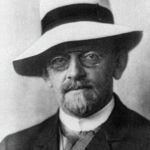David Hilbert#32
Most Influential Person

German mathematician

Why Is David Hilbert Influential?

According to Wikipedia, David Hilbert was a German mathematician and one of the most influential mathematicians of the 19th and early 20th centuries. Hilbert discovered and developed a broad range of fundamental ideas in many areas, including invariant theory, the calculus of variations, commutative algebra, algebraic number theory, the foundations of geometry, spectral theory of operators and its application to integral equations, mathematical physics, and the foundations of mathematics .

David Hilbert's Published Works